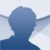# Math Formulary Android

3.0.26

## Math Formulary is an app that gathers the most important maths formulas of different areas, such as algebra, arithmetics or geometry, among others

Are you a student and you use many formulas in your daily work? You can now carry around the most important formulas on your Android mobile to be able to look them up at any moment. Download Math Formulary for free and you will have the formulas of different areas only one click away:

• Basic arithmetics
• Algebra
• Analysis
• Geometry
• Trigonometry
• Analytical geometry
• Logic

Maths formulas that can be needed at school or university.

With Math Formulary you won't need to resort to your paper or digital notes to find the formula you are searching for. Solve your problems by downloading Math Formulary free for Android.